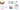# Class 10 Chapter 14 Statistics Application of Mean , Median and Mode

Posted on August 14th, 2018

## Class 10 Chapter 14 Statistics Application of mean, median and mode

In this article, we will discuss Chapter 14 Statistics – Application of mean,median and mode for Class 10 maths.

Statistics

Statistics is the science of average & estimates.  It is the study of collection, analysis, interpretation & Organization of data for specific purpose. We take decision with that data.Data : Everyday, we come across a wide variety of information in the form of facts, numerical figure, table groups etc. Eg: Information related to profit/ loss of a company, average cricket score for a country, Government expenditure in various sector in a given year, election results, Disease vitality information, Movie collection etc. This information is generally provided by TV, magazine, radio, internet etc.  These facts or figure which is numerical or otherwise, collected with a definite purpose is called data. This word is derived by Latin word Datum

Usage of statistics:

1. Demography data
2. Sales trends
3. Un-employement ratio
4. Data for business decision
5. Experimental data### Application of Mean, Median & Mode

1. Mean, median & mode shows different perspective of same data.
2. Mean gives average of the data. All data is given equal importance. It is used in case where all data is important. E.g. Average salary of employees in an organization
3. Median is used to find middle most data. It is used to determine a point from where 50% of data is more & 50% data is less. It is used where extreme cases can be ignored. E.g. To find the performance of a cricketer where his worst & best extreme performance can be ignored to give his consistent performance.
4. Mode is used where we need to find the most frequent data. E.g. if we need to find the most favorite Subject of students in a given class, mode can be used.

Flash back from IX Class

A systematic record of facts or different values of a quantity is called data.

### Features of the data :

• Statistics deals with collection, presentation, analysis and interpretation of numerical data.
• Arranging data in a order to study their salient features is called presentation of data.
• Data arranged in ascending or descending order is called arrayed data or an array
• Range of the data is the difference between the maximum and the minimum values of the observations
• Table that shows the frequency of different values in the given data is called a frequency distribution table
• A frequency distribution table that shows the frequency of each individual value in the given data is called an ungrouped frequency distribution table.
• A table that shows the frequency of groups of values in the given data is called a grouped frequency distribution table
• The groupings used to group the values in given data are called classes or class-intervals. The number of values that each class contains is called the class size or class width. The lower value in a class is called the lower class limit. The higher value in a class is called the upper class limit.
• Class mark of a class is the mid value of the two limits of that class.
• A frequency distribution in which the upper limit of one class differs from the lower limit of the succeeding class is called an Inclusive or discontinuous Frequency Distribution.
• A frequency distribution in which the upper limit of one class coincides from the lower limit of the succeeding class is called an exclusive or continuous Frequency Distribution

Bar Graph:

A bar graph is a pictorial representation of data in which rectangular bars of uniform width are drawn with equal spacing between them on one axis, usually the x axis. The value of the variable is shown on the other axis that is the y axis.

Histogram:

A histogram is a set of adjacent rectangles whose areas are proportional to the frequencies of a given continuous frequency distribution

Mean

Median

The median of a set of data values is the middle value of the data set when it has been arranged in ascending order.  That is, from the smallest value to the highest value
Median is calculated as

Where n is the number of values in the data. If the number of values in the data set is even, then the median is the average of the two middle value

Mode

Mode of a statistical data is the value of that variable which has the maximum frequency.

Get class 10 maths online classes at Takshila Learning.

Here at Takshila Learning, we provide Online Tuition Classes from Preschool to Class 12 for all subjects. We provide the best online tuition classes that comprise Animated Video lectures and Live Interactive Classes that will help students to grasp concepts easily.

We also provide Worksheets & Assignments, Doubt sessions, Free Encyclopedia, NCERT Solutions, and Exam Guru to monitor your progress, i.e., subject-wise and topic-wise. So now Enjoy E-Learning with Takshila Learning…

Learning is fun now!
Enroll today & Get Free Demo Class!

## Call at 8800999280 / 8800999283 / 8800999284 fill the form for any other details:

Important Tags : Class 10 Chapter 14 Statistics Application of Mean, Application of Median , Application of Mode , Class 10 Application of Mean , Class 10 Application of Median

0Shares